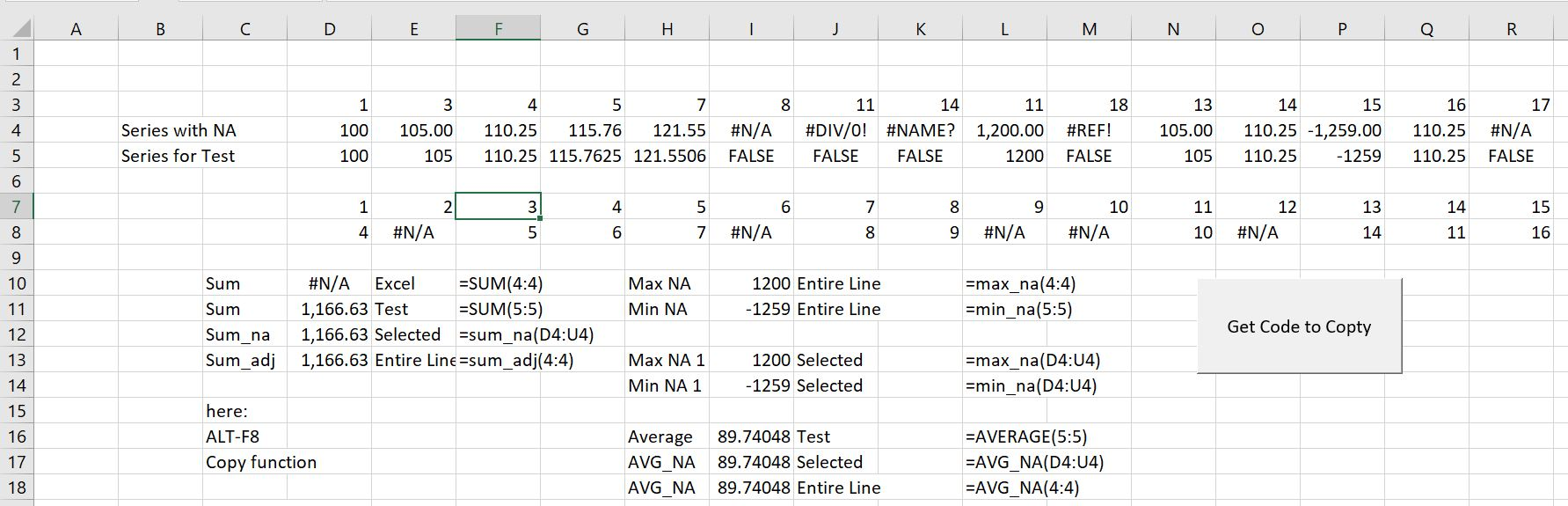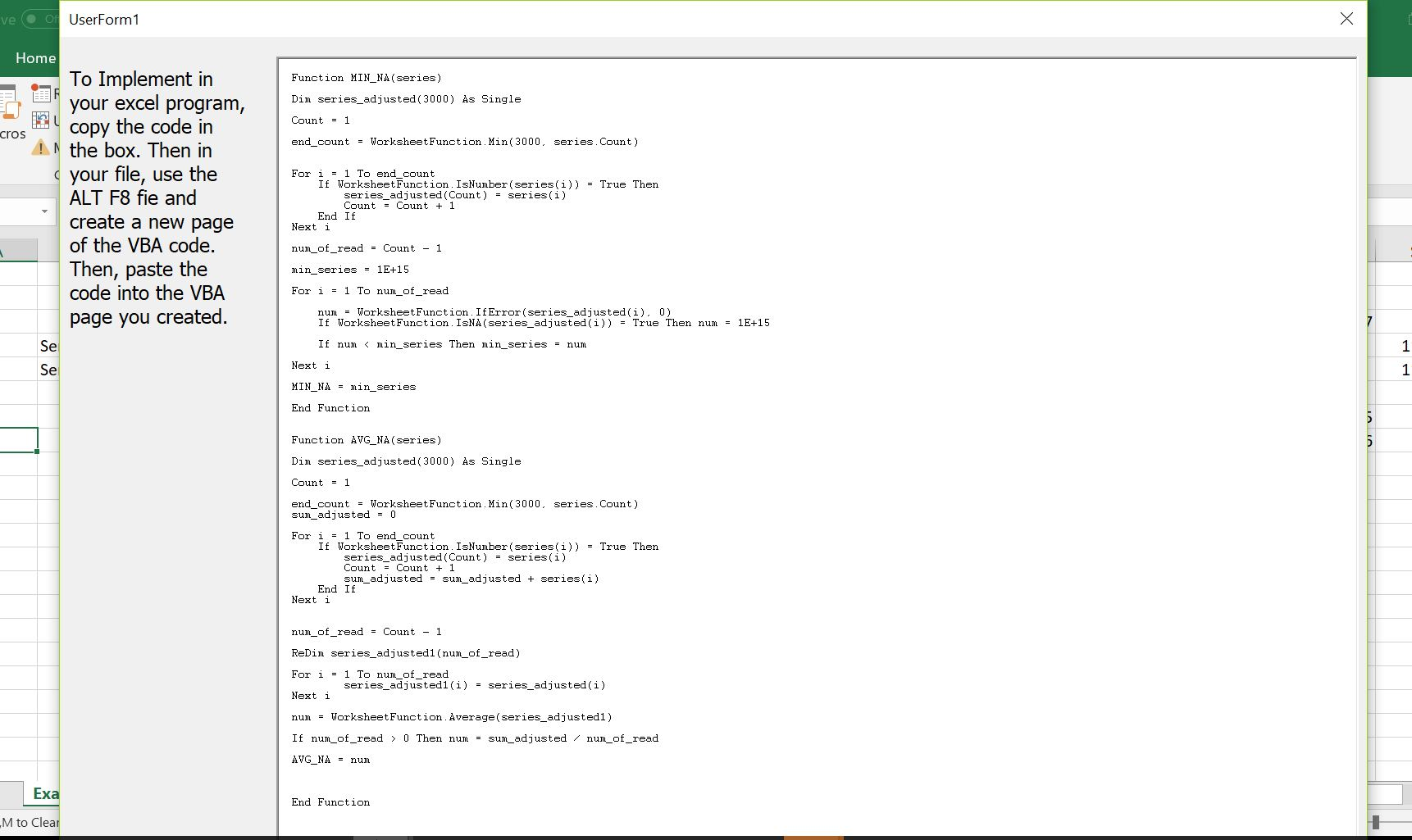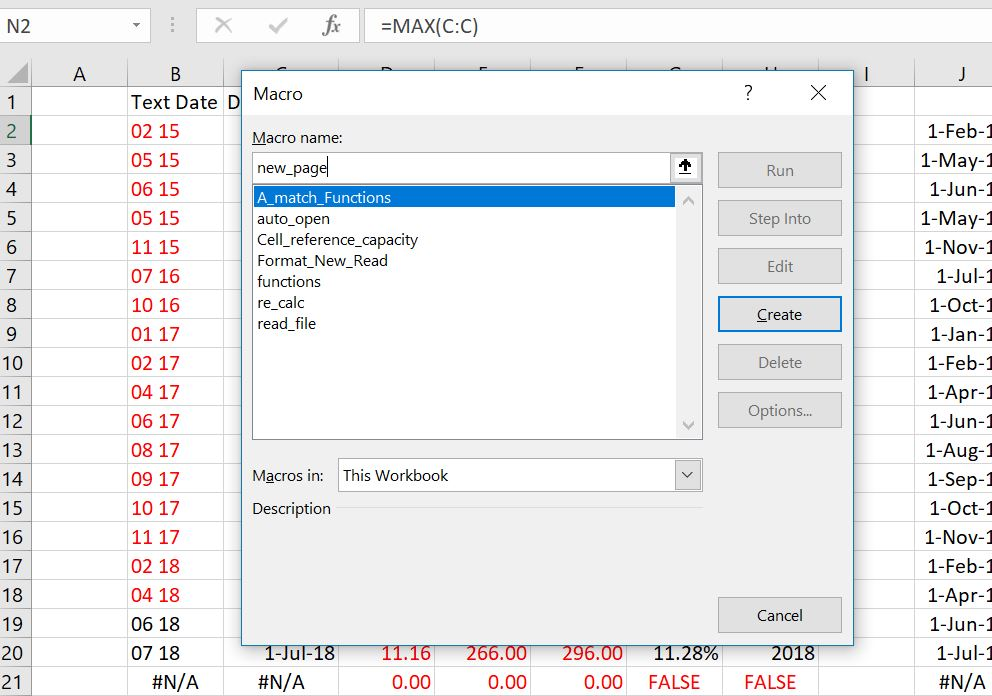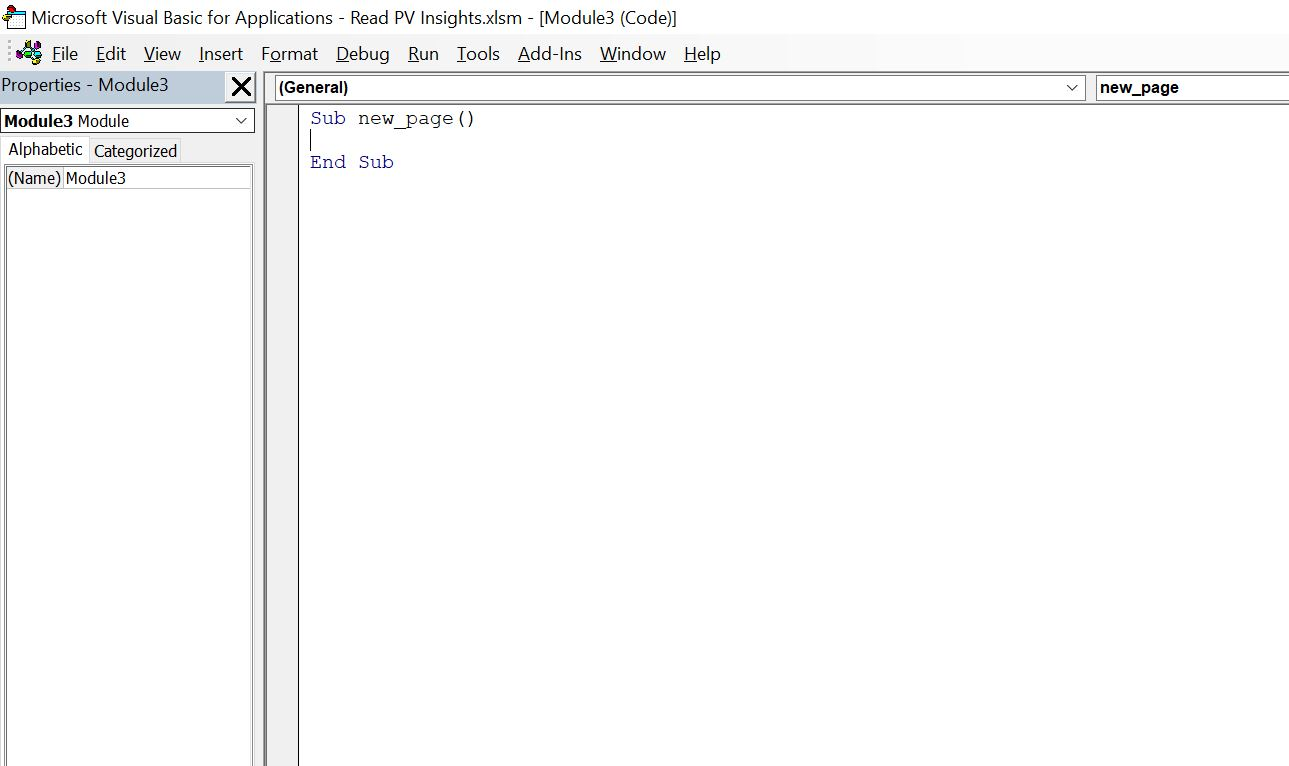# Sum #NA etc.

This page demonstrates how to create user defined functions that adjust the sum, average, max and min functions to work when there are #N/A, VALUE!, #NAME? and #REF! values in the series.  If a series has 100, #N/A, 200, 300 and you try and compute the sum or average etc., the function will not work.  User defined functions discussed on this page compute the sum and average etc. through ignoring the non-numbers.

The functions that fix the various excel functions are included in the excel file that you can download by pressing the button below. This page explains how to use the file to copy the adjusted SUM, MAX,MIN and AVERAGE functions into your file.

The screenshot below illustrates the issue that is resolved by these functions.  The first line on row 4 has numbers with various problems including #N/A, #DIV/0! etc.  If you use functions to compute the sum, maximum, minimum etc. The data on line 5 is repaired using FALSE and ISNUMBER function.  The SUM_NA, MAX_NA, MIN_NA, and AVERAGE_NA functions ignore the problematic data.When you open the file, a screen appears with a box that allows you to copy the VBA code for the functions. Once you have copied the functions, you can paste it into a VBA module of your file.  You can also copy the code from the screen that appears when you open the file or you can press the button named “Get Code to Copy” shown in the screenshot above.  When the file opens or when you press the button, a screen appears like the one shown in the screenshot below.  In the screenshot below, the area in the box should be selected (CNTL, A) and the CNTL, C can be pressed to copy.The second step, once you have copied the code from the box in the form that appears, is to copy the code into a VBA module in your file.  To do this, press the ALT, F8 sequence to see the list of current macros.  Then type some random macro name to create a new temporary program that will leave space for your copied function.  Note two things.  First, you have to copy the function code into your sheet and you cannot just access it from another file as with other macros that are subroutines and require some kind of action to complete.  Second, the name of the user defined function does not appear in the list of macros. After creating a blank VBA page, with the macro name, insert a few lines so the code that you copy goes to the top of the file. The process of pressing ALT, F8 and then typing a new macro name is illustrated in the screenshot below.The next screenshot simply illustrates what happens after you enter your new name and a blank macro appears.  You will do nothing with this macro and just insert the code that you copied in step 1 to this page.The next screenshot simply demonstrates that you should make space for copying the code into your file. Often with the functions that I use, you need to copy the code to the top of the page because I apply the Option Base 1 statement.The final screen shot just demonstrates the result of copying the file. Note that the statement Option Base 1 is at the top of the page.  This option is used to begin counting array variables with 1 instead of 0.## VBA Code for SUM_NA, MAX_NA, MIN_NA, and AVERAGE_NA

The code below shows how I have programmed the functions. The first part of the function is designed so that you can use an entire row or column.  In this case with the entire line, I re-define a series that does not include any blanks or non-numeric items.

.

```Option Base 1
Sub functions_sumNA_Max_NA()

End Sub

Count = 1

end_count = WorksheetFunction.Min(3000, series.Count)

For i = 1 To end_count
If WorksheetFunction.IsNumber(series(i)) = True Then
Count = Count + 1
End If
Next i

For i = 1 To num_of_read

Next i

End Function

Function SUM_NA(series)

Count = 1

end_count = WorksheetFunction.Min(3000, series.Count)

For i = 1 To end_count
If WorksheetFunction.IsNumber(series(i)) = True Then
Count = Count + 1
End If
Next i

For i = 1 To num_of_read

If WorksheetFunction.IsNA(series_adjusted(i)) = True Then num = 0

SUM_NA = SUM_NA + num

Next i

End Function

Function MAX_NA(series)

Count = 1

end_count = WorksheetFunction.Min(3000, series.Count)

For i = 1 To end_count
' MsgBox " i " & i & " series(i) " & series(i)

On Error Resume Next

If WorksheetFunction.IsNumber(series(i)) = True Then
Count = Count + 1
End If
Next i

max_series = -1E+15

For i = 1 To num_of_read

If WorksheetFunction.IsNA(series_adjusted(i)) = True Then num = -1E+15

If num > max_series Then max_series = num

Next i

MAX_NA = max_series

End Function

Function MIN_NA(series)

Count = 1

end_count = WorksheetFunction.Min(3000, series.Count)

For i = 1 To end_count
If WorksheetFunction.IsNumber(series(i)) = True Then
Count = Count + 1
End If
Next i

min_series = 1E+15

For i = 1 To num_of_read

If WorksheetFunction.IsNA(series_adjusted(i)) = True Then num = 1E+15

If num < min_series Then min_series = num

Next i

MIN_NA = min_series

End Function

Function AVG_NA(series)

Count = 1

end_count = WorksheetFunction.Min(3000, series.Count)

For i = 1 To end_count
If WorksheetFunction.IsNumber(series(i)) = True Then
Count = Count + 1
End If
Next i

For i = 1 To num_of_read
Next i

AVG_NA = num

End Function

```